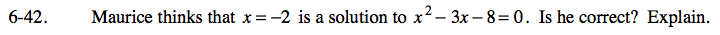### Home > CAAC > Chapter 6 > Lesson 6.2.1 > Problem6-42

6-42.

Maurice thinks that x = −2 is a solution to x2 − 3x − 8 = 0. Is he correct? Explain. Homework Help ✎Substitute −2 into the equation for x and see if it makes the equation true.

No, −2 is not a solution to this equation.